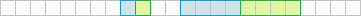#Boost C++ Libraries

...one of the most highly regarded and expertly designed C++ library projects in the world.

### Performance considerations

Technical details aside, the memory layout of `optional<T>` is more-less this:

```template <typename T>
class optional
{
bool _initialized;
std::aligned_storage_t<sizeof(t), alignof(T)> _storage;
};
```

But for the purpose of this analysis, considering memory layouts, we can think of it as:

```template <typename T>
class optional
{
bool _initialized;
T _storage;
};
```

Given type `optional<int>`, and assuming that ```sizeof(int) == 4```, we will get ```sizeof(optional<int>) == 8```. This is so because of the alignment rules, for our two members we get the following alignment:This means you can fit twice as many `int`s as `optional<int>`s into the same space of memory. Therefore, if the size of the objects is critical for your application (e.g., because you want to utilize your CPU cache in order to gain performance) and you have determined you are willing to trade the code clarity, it is recommended that you simply go with type `int` and use some 'magic value' to represent not-an-int.

Even if you cannot spare any value of `int` to represent not-an-int (e.g., because every value is useful, or you do want to signal not-an-int explicitly), at least for `Trivial` types you should consider storing the value and the `bool` flag representing the null-state separately. Consider the following class:

```struct Record
{
optional<int> _min;
optional<int> _max;
};
```

Its memory layout can be depicted as follows:This is exactly the same as if we had the following members:

```struct Record
{
bool _has_min;
int  _min;
bool _has_max;
int  _max;
};
```

But when they are stored separately, we at least have an option to reorder them like this:

```struct Record
{
bool _has_min;
bool _has_max;
int  _min;
int  _max;
};
```

Which gives us the following layout (and smaller total size):Sometimes it requires detailed consideration what data we make optional. In our case above, if we determine that both minimum and maximum value can be provided or not provided together, but one is never provided without the other, we can make only one optional memebr:

```struct Limits
{
int  _min;
int  _max;
};

struct Record
{
optional<Limits> _limits;
};
```

This would give us the following layout:##### Optional function parameters

Having function parameters of type ```const optional<T>&``` may incur certain unexpected run-time cost connected to copy construction of `T`. Consider the following code.

```void fun(const optional<Big>& v)
{
if (v) doSomethingWith(*v);
else   doSomethingElse();
}

int main()
{
optional<Big> ov;
Big v;
fun(none);
fun(ov); // no copy
fun(v);  // copy constructor of Big
}
```

No copy elision or move semantics can save us from copying type `Big` here. Not that we need any copy, but this is how `optional` works. In order to avoid copying in this case, one could provide second overload of `fun`:

```void fun(const Big& v)
{
doSomethingWith(v);
}

int main()
{
optional<Big> ov;
Big v;
fun(ov); // no copy
fun(v);  // no copy: second overload selected
}
```

Alternatively, you could consider using an optional reference instead:

```void fun(optional<const Big&> v) // note where the reference is
{
if (v) doSomethingWith(*v);
else   doSomethingElse();
}

int main()
{
optional<Big> ov;
Big v;
fun(none);
fun(ov); // doesn't compile
fun(v);  // no copy
}
```